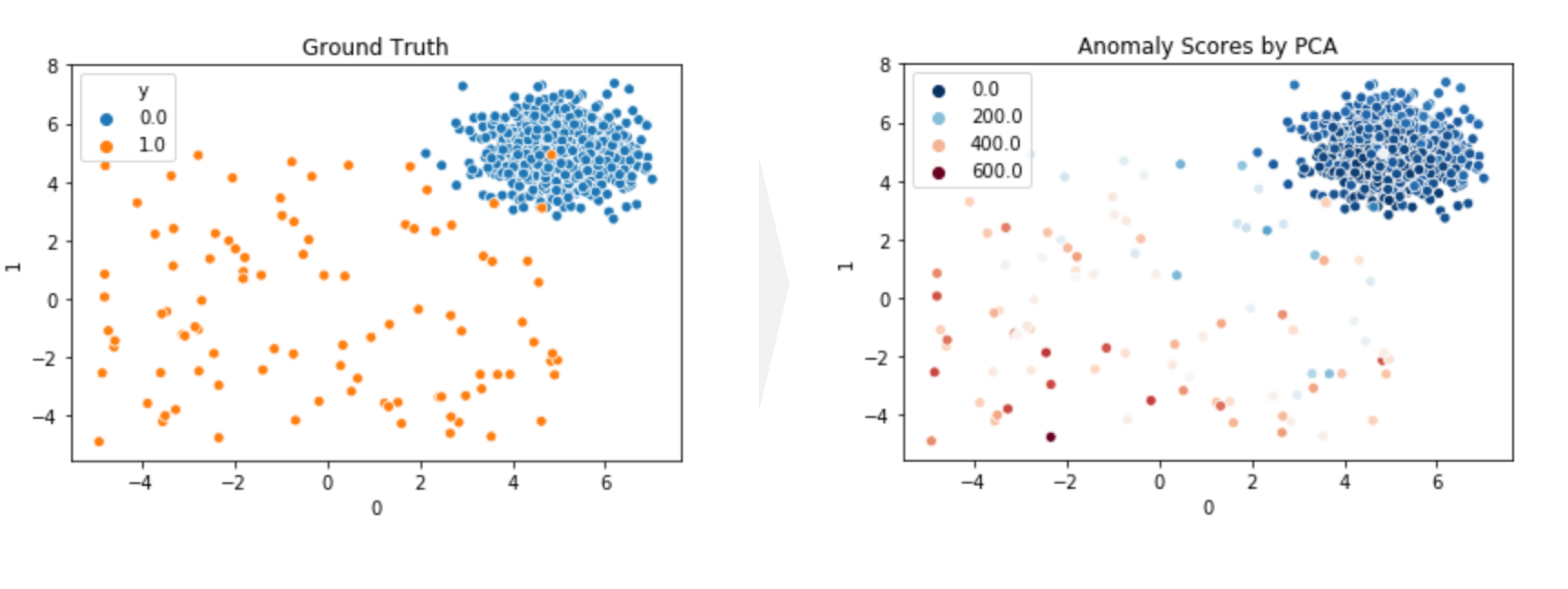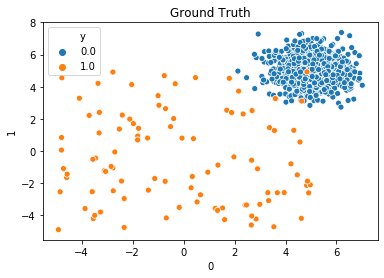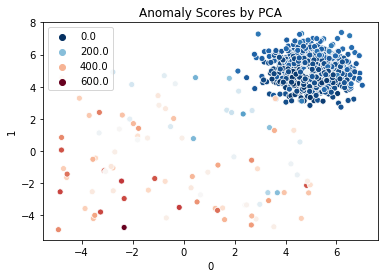# Anomaly Detection by PCA in PyOD

## Goal¶

This post aims to introduce how to detect anomaly using PCA in pyod.Reference

## Libraries¶

In :
import pandas as pd
import numpy as np
import matplotlib.pyplot as plt
import seaborn as sns
%matplotlib inline

# PyOD
from pyod.utils.data import generate_data, get_outliers_inliers
from pyod.models.pca import PCA
from pyod.utils.data import evaluate_print
from pyod.utils.example import visualize


## Create a data¶

In :
X_train, y_train = generate_data(behaviour='new', n_features=5, train_only=True)
df_train = pd.DataFrame(X_train)
df_train['y'] = y_train

In :
df_train.head()

Out:
0 1 2 3 4 y
0 5.475324 4.882372 5.337351 5.376340 4.104947 0.0
1 5.244566 5.626358 5.356578 4.341500 4.856838 0.0
2 4.597031 5.787669 5.959738 5.823086 6.012408 0.0
3 4.637728 4.639901 5.400144 6.074926 4.627883 0.0
4 4.639908 4.667926 6.077212 5.012901 3.718718 0.0
In :
sns.scatterplot(x=0, y=1, hue='y', data=df_train);
plt.title('Ground Truth');## Train an unsupervised PCA¶

In :
clf = PCA()
clf.fit(X_train)

Out:
PCA(contamination=0.1, copy=True, iterated_power='auto', n_components=None,
n_selected_components=None, random_state=None, standardization=True,
svd_solver='auto', tol=0.0, weighted=True, whiten=False)

## Evaluate training score¶

In :
y_train_pred = clf.labels_
y_train_scores = clf.decision_scores_
sns.scatterplot(x=0, y=1, hue=y_train_scores, data=df_train, palette='RdBu_r');
plt.title('Anomaly Scores by PCA');